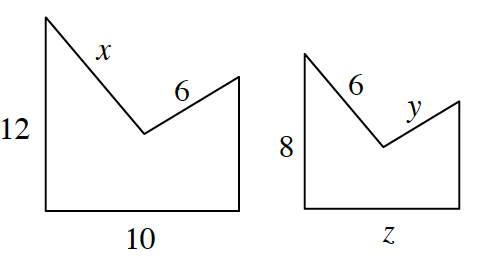### Home > CCG > Chapter 4 > Lesson 4.1.1 > Problem4-9

4-9.Assume that the shapes at right are similar. Find the values of $x$, $y$, and $z$.

For each of the variables, set up equivalent ratios with corresponding sides of known value.

Several ratios are possible, especially as the lengths of additional sides are found.

$\frac{x}{12}=\frac{6}{8}$

Solve for $x$ by first multiplying both sides of the equation by the lowest common denominator.